# Autotelic Computing

## Tuesday, June 17, 2014

### DBSCAN Blues

My previous machine learning posts:

Suppose I give you this dataset of 2D points (with the added twist that the generating code has been obfuscated, to avoid making the answer too obvious), and ask you to group them into $k$ meaningful clusters. Is there a way to do it without knowing (or guessing) $k$ in advance, as $k$-means would require? Yes, with the DBSCAN algorithm, but at the expense of introducing two additional parameters! In this post, I try to show why it's not as bad as it may sound by first describing the intuition behind DBSCAN, fiddling next with the problem of parameter estimation and finally showing an equivalent way of looking at it, in terms of graph operations.

In :
import base64
random.seed(0)
# this obfuscated code just defines a 'points' dataset, as a numpy array
obfuscated_code = ('cG9pbnRzMCA9IHJhbmRvbS5tdWx0aXZhcmlhdGVfbm9ybWFs'
'KFswLCAwXSwgZXllKDIpLCAzMDApCnBvaW50czEgPSByYW5k'
'b20ubXVsdGl2YXJpYXRlX25vcm1hbChbNSwgNV0sIGV5ZSgy'
'KSArIDIsIDMwMCkKcG9pbnRzMiA9IHJhbmRvbS5tdWx0aXZh'
'cmlhdGVfbm9ybWFsKFs4LCAyXSwgZXllKDIpLCAzMDApCnBv'
'aW50cyA9IHZzdGFjaygocG9pbnRzMCwgcG9pbnRzMSwgcG9p'
'bnRzMikp')
eval(compile(base64.b64decode(obfuscated_code), '<string>','exec'))
# 'points' is defined at this point
random.shuffle(points)
scatter(*zip(*points), color=[.5] * 3, s=5);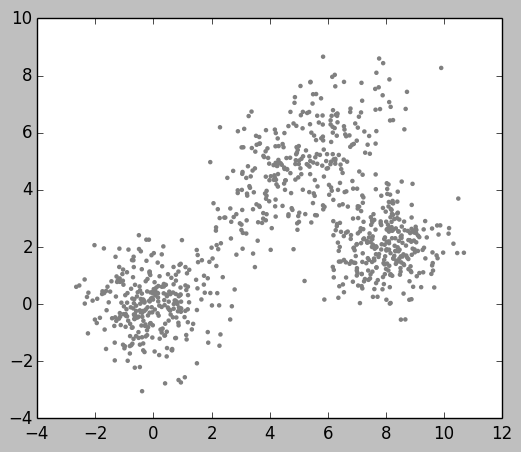Like $k$-means, DBSCAN is quite a simple idea. But to be honest, I find the formal introduction (from the Wikipedia article) slightly confusing, whereas the basic idea is really simple: a point is included in a cluster if either:

1. It's surrounded by at least a certain number of neighbors inside a certain radius.
2. It's inside a certain distance of a point as defined in 1.

Let's call the first type core points, and the second reachable points. The remaining points (if any) are noise, and we don't care about them (contrary to $k$-means, DBSCAN can easily result in uncategorized points, which can be meaningful for certain applications).

So first things first, if we are to implement an algorithm based on the notions of distance and neighborhoods, we'd better have at our disposal an efficient way of retrieving the nearest neighbors of a point in terms of a certain distance metric. The naive way of doing it (computing every pairwise possibilities) would be $O(n^2)$, but it turns out that for relatively low-dimensional (i.e. < 20-ish) Euclidean spaces, a k-d tree is a very reasonable and efficient way of doing it. So here's how to use a scipy.cKDTree to retrieve all the neighbors inside a radius of 1 of the first dataset point:

In :
import scipy.spatial
tree = scipy.spatial.cKDTree(points)
neighbors = tree.query_ball_point(points, 1)
scatter(*zip(*points), color=[.75] * 3, s=2)
scatter(*zip(*points[neighbors]), color='r', s=5)
scatter(*points, color='b', s=5)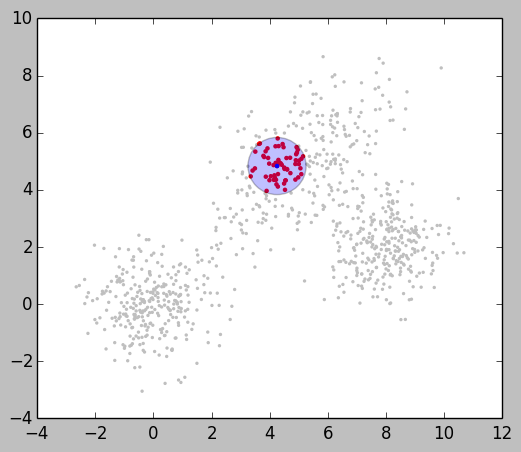We now have everything we need to define and implement the DBSCAN algorithm. Although Python is itself stylistiscally very close to pseudocode, the essence of the algorithm can be summarized in words as: for every unvisited point with enough neighbors, start a cluster by adding them all in, and then, for each, recursively expand the cluster if they also have enough neighbors, and stop expanding otherwise. Although I used the word "recursively", you'll notice that our implementation is actually not:

In :
def DBSCAN(points, eps, min_pts):

tree = scipy.spatial.cKDTree(points)
neighbors = tree.query_ball_point(points, eps)
clusters = [] # list of (set, set)'s, to distinguish
# core/reachable points
visited = set()
for i in xrange(len(points)):
if i in visited:
continue
if len(neighbors[i]) >= min_pts:
clusters.append(({i}, set())) # core
to_merge_in_cluster = set(neighbors[i])
while to_merge_in_cluster:
j = to_merge_in_cluster.pop()
if j not in visited:
if len(neighbors[j]) >= min_pts:
to_merge_in_cluster |= set(neighbors[j])
if not any([j in c | c for c in clusters]):
if len(neighbors[j]) >= min_pts:
else:
return clusters


Now that we have an algorithm, you may ask a very legitimate question: how do we choose eps (the minimal distance/radius) and min_pts (the minimal number of points inside that radius to start/expand a cluster)? If we pick an arbitrary value for min_pts (say 10), we could try looking at the distribution of distances separating every point from their 10 nearest neighbors:

In :
min_pts = 10
nn_dists = ravel(tree.query(points, k=min_pts + 1)[:, 1:])
assert len(nn_dists) == len(points) * min_pts
hist(nn_dists, bins=100);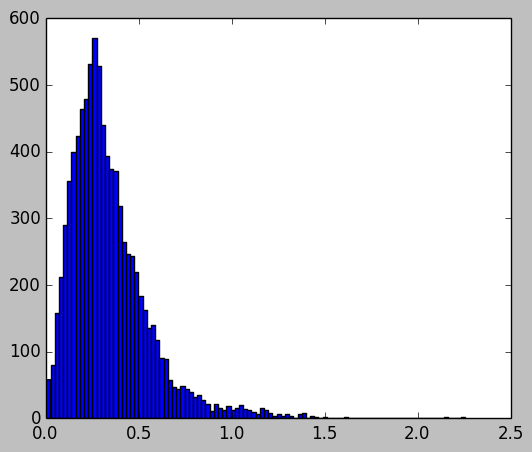So it would seem that a value of eps somewhere between 0.25 and 1 would make sense.. let's try:

In :
clusters = DBSCAN(points, 0.25, min_pts)

scatter(points[:, 0], points[:, 1], color=[.75] * 3, alpha=.5, s=5)
colors = 'rbgycm' * 10
for i, (core, reachable) in enumerate(clusters):
core = list(core); reachable = list(reachable)
scatter(*zip(*points[core]), color=colors[i], alpha=1, s=5)
scatter(*zip(*points[reachable]), color=colors[i], alpha=.4, s=5)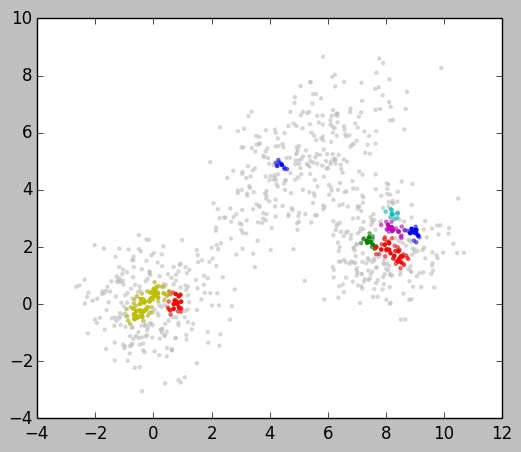Clearly not working very well.. I'm a somewhat suspicious coder: could it be a bug with our implementation of DBSCAN? To verify, let's try scikit-learn's version, which is certainly right:

In :
import sklearn.cluster

clusters = sklearn.cluster.DBSCAN(0.25, min_pts).fit_predict(points)

scatter(points[:,0], points[:,1], color=[.75] * 3, alpha=.5, s=5)
for i in set(clusters):
if i < 0: continue
scatter(*zip(*points[where(clusters == i)]),
color=colors[int(i)], alpha=1, s=5)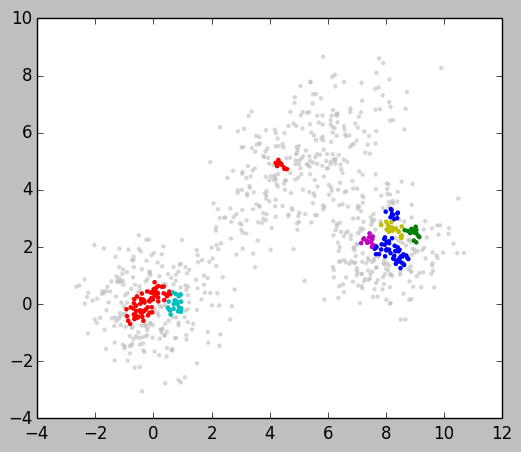Identical results (apart from the color code).. which spells good news for our implementation, but bad news for our choice of parameters.. let's see what happens with eps = 0.5:

In :
clusters = DBSCAN(points, 0.5, min_pts)

scatter(*zip(*points), color=[.75] * 3, alpha=.5, s=5)
for i, (core, reachable) in enumerate(clusters):
core = list(core); reachable = list(reachable)
scatter(*zip(*points[core]), color=colors[i], alpha=1, s=5)
scatter(*zip(*points[reachable]), color=colors[i], alpha=.4, s=5)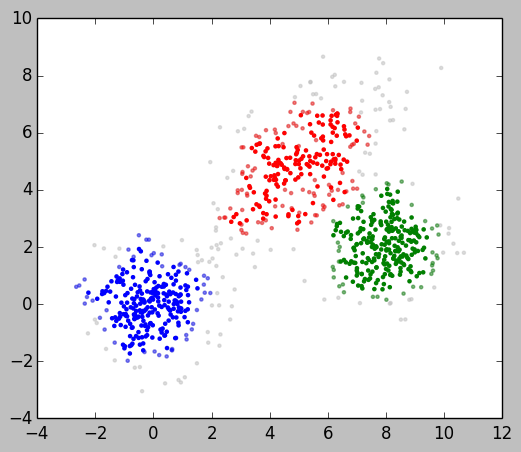All right, much better (also note the graphical distinction between core and reachable points, the latter being a bit paler)! And it wasn't that hard to come up with reasonable parameter values.. but are we really convinced yet that DBSCAN is such an improvement over $k$-means? Picking a value for min_pts was still pretty much arbitrary.. To continue our exploration, let's create a dataset which would be very hard (in fact impossible) for $k$-means to make sense of:

In :
inner = random.multivariate_normal([0, 0], eye(2), 500)
rr, rn = random.random, random.normal
outer = [(r * cos(t), r * sin(t))
for r, t in [(rn(3.5, 0.25), 2 * pi * rr())
for _ in range(500)]]
points2 = vstack((inner, outer))
random.shuffle(points2)
scatter(*zip(*points2), color=[.4] * 3, s=5);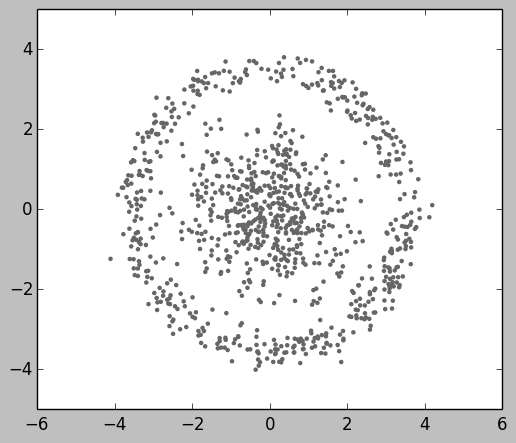In :
clusters = DBSCAN(points2, 0.5, min_pts)

scatter(*zip(*points2), color=[.75] * 3, alpha=.5, s=5)
for i, (core, reachable) in enumerate(clusters):
core = list(core); reachable = list(reachable)
scatter(*zip(*points2[core]), color=colors[i], alpha=1, s=5)
scatter(*zip(*points2[reachable]), color=colors[i], alpha=.4, s=5)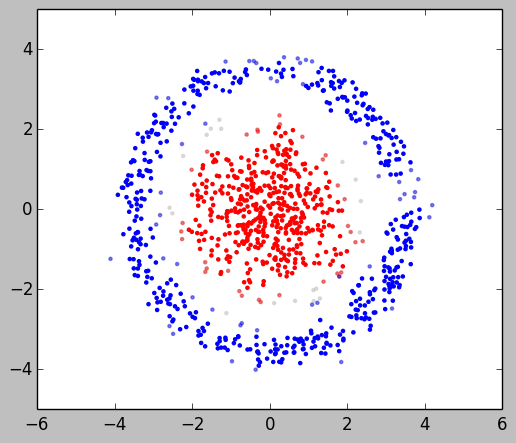Very reasonable and interesting results! To conclude, let's examine an alternative way of looking at the DBSCAN algorithm, from the perspective of graph theory. It turns out that DBSCAN is equivalent to finding the connected components of a graph defined as: "for every node, draw an edge to every neighbors inside a certain radius (eps), if they are in sufficient number (min_pts)". This is very easy to implement using the networkx library, and yields the expected results:

In :
import networkx as nx

neighbors = tree.query_ball_point(points, 0.5)

g = nx.Graph()
nx.draw_networkx(g, points, with_labels=False, node_size=5,
node_color=[.5] * 3, width=.5)
g = nx.Graph()
for i in xrange(len(points)):
if len(neighbors[i]) >= 10:
g.add_edges_from([(i, j) for j in neighbors[i]])

for i, comp in enumerate(nx.connected_component_subgraphs(g)):
nx.draw_networkx(comp, points, with_labels=False, node_size=5,
node_color=[.5] * 3, edge_color=colors[i], width=.5)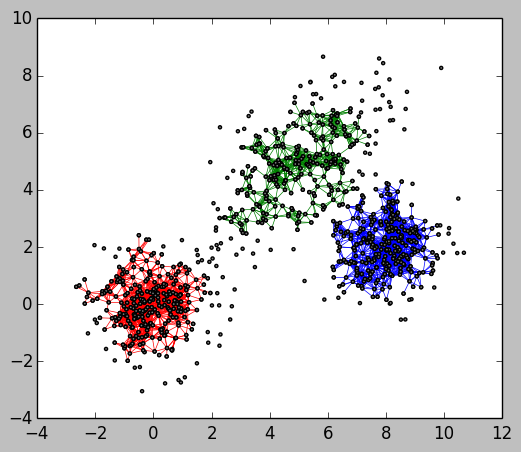Although interesting, this equivalence of course does not yield a more efficient algorithm, because it still relies on the neighborhood-finding helper (in our case a $k$-d tree), which dominates the overall complexity by being $O(n \log n)$.